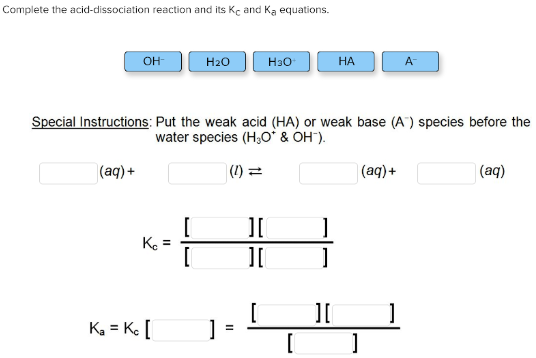# Complete the acid-dissociation reaction and its Kc and Ka equations. OH- H2O H3O+ HA A- Special instructions: Put the weak acid (HA) or weak base (A-) species before the water species (H3O+ & OH-) ___(aq) + ___(l) <-> ___(aq) + ___(aq) Kc = [__][__]/[__][__] Ka = Kc [__] = [__][__]/[__]